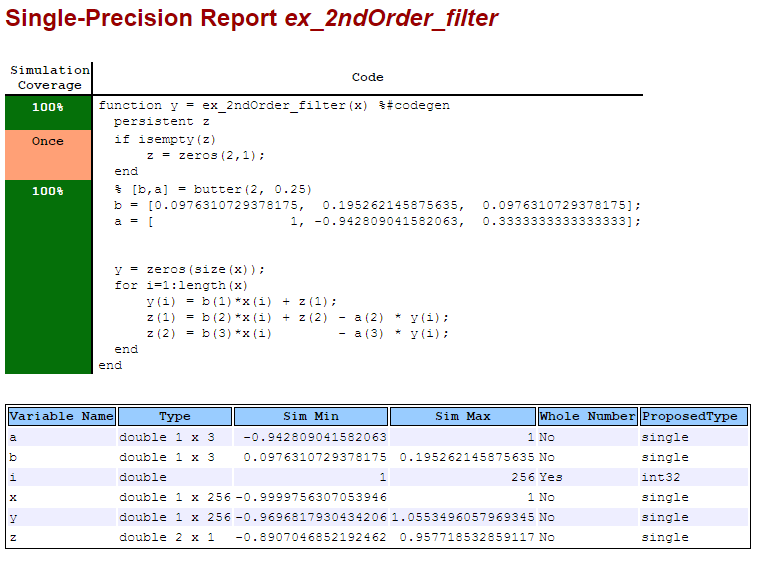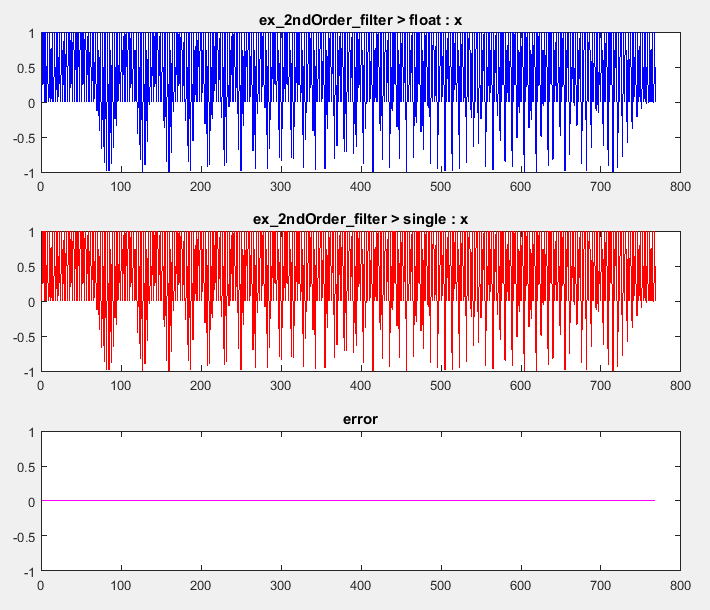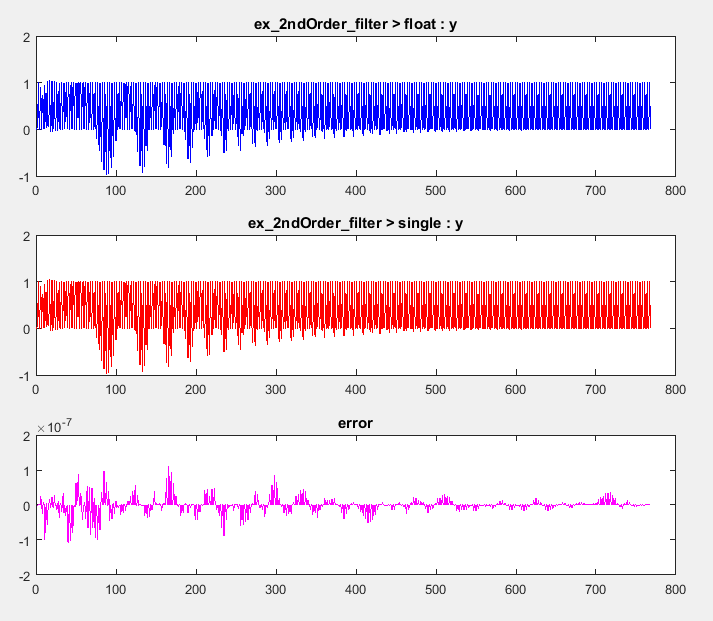## Generate Single-Precision MATLAB Code

This example shows how to generate single-precision MATLAB® code from double-precision MATLAB code. This example shows the single-precision conversion workflow that you use when you want to see single-precision MATLAB code or use verification options. Optionally, you can also generate single-precision C/C++ code.

### Prerequisites

To complete this example, install the following products:

• MATLAB

• MATLAB Coder™

• Fixed-Point Designer™

• C compiler

You can use `mex -setup` to change the default compiler. See Change Default Compiler (MATLAB).

### Create a Folder and Copy Relevant Files

1. Create a local working folder, for example, `c:\ex_2ndOrder_filter`.

2. Change to the `docroot\toolbox\fixpoint\examples` folder. At the MATLAB command line, enter:

`cd(fullfile(docroot, 'toolbox', 'fixpoint', 'examples')) `

3. Copy the `ex_2ndOrder_filter.m` and `ex_2ndOrder_filter_test.m` files to your local working folder.

TypeNameDescription
Function code`ex_2ndOrder_filter.m`Entry-point MATLAB function
Test file`ex_2ndOrder_filter_test.m`

MATLAB script that tests `ex_2ndOrder_filter.m`

### Set Up the Single-Precision Configuration Object

Create a single-precision configuration object. Specify the test file name. Verify the single-precision code using the test file. Plot the error between the double-precision code and single-precision code. Use the default values for the other properties.

```scfg = coder.config('single'); scfg.TestBenchName = 'ex_2ndOrder_filter_test'; scfg.TestNumerics = true; scfg.LogIOForComparisonPlotting = true;```

### Generate Single-Precision MATLAB Code

To convert the double-precision MATLAB function, `ex_2ndOrder_filter`, to single-precision MATLAB code, use the `codegen` function with the `-double2single` option.

`codegen -double2single scfg ex_2ndOrder_filter`

`codegen` analyzes the double-precision code. The conversion process infers types by running the test file because you did not specify the input types for the `ex_2ndOrder_filter` function. The conversion process selects single-precision types for the double-precision variables. It selects `int32` for index variables. When the conversion is complete, `codegen` generates a type proposal report.

### View the Type Proposal Report

To see the types that the conversion process selected for the variables, open the type proposal report for the `ex_2ndOrder_filter` function. Click the link `ex_2ndOrder_filter_report.html`.

The report opens in a web browser. The conversion process converted:

• Double-precision variables to `single`.

• The index `i` to `int32`. The conversion process casts index and dimension variables to `int32`.### View Generated Single-Precision MATLAB Code

To view the report for the generation of the single-precision MATLAB code, in the Command Window:

1. Scroll to the `Generate Single-Precision Code` step. Click the View report link.

2. In the MATLAB Source pane, click `ex_2ndOrder_filter_single`.

The code generation report displays the single-precision MATLAB code for `ex_2ndOrder_filter`.

### View Potential Data Type Issues

When you generate single-precision code, `codegen` enables highlighting of potential data type issues in code generation reports. If `codegen` cannot remove a double-precision operation, the report highlights the MATLAB expression that results in the operation. Click the Code Insights tab. The absence of potential data type issues indicates that no double-precision operations remain.

### Compare the Double-Precision and Single-Precision Variables

You can see the comparison plots for the input `x` and output `y` because you selected to log inputs and outputs for comparison plots .### Optionally Generate Single-Precision C Code

If you also want to generate single-precision C code, create a code configuration object for C code generation. Use this configuration object with the `-config` option of the `codegen` function. For example:

1. Create a code configuration object for generation of a C static library.

```cfg = coder.config('lib'); ```

2. Generate the C code. Enable generation of the code generation report.

`codegen -double2single scfg -config cfg ex_2ndOrder_filter -report`
3. To view the code generation report for the C code generation, click the View Report link.

In the Generated Code pane, click `ex_2ndOrder_filter.c`.

• Double-precision variables have type `float` in the C code.

• The index `i` is an integer.

When you generate single-precision code, `codegen` enables highlighting of potential data type issues in the code generation report. If `codegen` cannot remove a double-precision operation, the report highlights the MATLAB expression that results in the operation.

Click the Code Insights tab. Then, expand Potential data type issues. The absence of double-precision operations indicates that no double-precision operations remain.